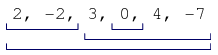Vaughan Reid's blog

# Code challenges: Finding number of sub arrays with a sum of zero

It can be fun to challenge yourself with algorithm type problems and to try find an optimal solution. Unfortunately the only way to get better at them is to practice. After enough practice you might start to spot common patterns to solve a new problem just based off experience.

I challenged myself to solve the following:

Find the number of fragments of an array that sum is equals to 0 (that is, pairs(P,Q) such that P’<”Q and the sum A[P]+A[P+1]…+A[Q] equals to 0).

For example: [-2,2,3,0,4,-7] returns 4 asAdditionally, an array with all zeros at max size of 10000 will return -1. Try find the optimal solution.

Personally, if I can use my own IDE, I will always start with unit tests. These will help validate my answer against the test cases as I look for edge cases.

``````[TestFixture]
public class ZeroFragmentsTests
{
[Test]
public void Example1()
{
int[] A = new int[] { 2, -2, 3, 0, 4, -7 };
int expected = 4;

int actual = new ZeroFragments().solution(A);

Assert.AreEqual(expected, actual);
}

[Test]
public void Example2()
{
int[] A = new int;
for (int i = 0; i < A.Length; i++) A[i] = 0;

int expected = -1;

int actual = new ZeroFragments().solution(A);

Assert.AreEqual(expected, actual);
}

[Test]
public void Example3()
{
int[] A = new int[] {6, 3, -1, -3, 4, -2, 2, 4, 6, -12, -7};
int expected = 5;

int actual = new ZeroFragments().solution(A);

Assert.AreEqual(expected, actual);
}
}

``````

Then unless the optimized answer is obvious, I will first try solve it with brute force. In this case, with 2 nested for-loops its O(n2) time complexity.

``````
private const int MaxTotal = 1000000000;

public int solution(int[] A)
{
int totalCount = 0;

for (int i = 0; i < A.Length; i++)
{
int sum = 0;

for (int j = i; j < A.Length; j++)
{
int num = A[j];
sum += num;
if (sum == 0)
{
totalCount++;
if (totalCount >= MaxTotal)
{
return -1;
}
}
}
}

}

``````

Whats great about a brute force approach first is that you can often create a few edge case unit tests to make sure any future improvements still behave correctly.

The question specifically asked for an optimized solution and so that is what I will do! An optimized solution only makes sense if you are expecting large arrays. At that point, time complexity of your algorithm will make a difference.

Generally when you are at O(n2) and need to optimized, the first question I will ask is if the divide and conquer approach will work? If I break the array into sub parts, can I solve each part and then add up the result. With that you would be looking at O(n*logn) which is much better. To be honest I don’t know if this solution can work with divide and conquer because I’m not sure how it would handle sums across sub arrays without doing something closer to the brute force approach again.

The next approach is to try understand the data and hopefully get an insight that will simplify it. In this case, that is the way that works. The key insight is that instead of looking at a sample array as it is.

[4, -5, 5]

Rather look at it as an array of the cumulative sums.

[4, 4-5, 4-5+5] = [4, -1, 4]

Maybe its just me but if I think of each index as a height, then intuitively if another index is at the same height then there has been a zero sum of heights between the points.

Maybe not the best explanation but by using that insight, you only need to go through the array once with a dictionary tracking where the sums matches. So amazingly you can change the O(n^2) into O(n).

``````
public class ZeroFragments
{
private const int MaxTotal = 1000000000;

public int solution(int[] A)
{
int totalCount = 0 ;
int sum = 0;
IDictionary<int, int> cumulative = new Dictionary<int, int>();

for (int i = 0; i < A.Length; i++)
{
int num = A[i];

sum += num;

if (sum == 0)
{
totalCount++;
}

if(cumulative.TryGetValue(sum, out var prevCount))
{
totalCount += prevCount++;
}
else
{
prevCount++;
}
cumulative[sum] = prevCount;

if (totalCount >= MaxTotal)
{
return -1;
}
}